International Journal of Energy and Power Engineering
Volume 5, Issue 2, April 2016, Pages: 39-47

Prandtl Number Effect of Mixed Convection Heat and Mass Transfer in a Triangular Enclosure with Heated Circular Obstacle

Sayeda Fahmida Ferdousi1, 2,*, Md. Abdul Alim2, Raju Chowdhury1, 2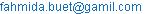(S. F. Ferdousi)

*Corresponding author

Sayeda Fahmida Ferdousi, Md. Abdul Alim, Raju Chowdhury. Prandtl Number Effect of Mixed Convection Heat and Mass Transfer in a Triangular Enclosure with Heated Circular Obstacle. International Journal of Energy and Power Engineering. Vol. 5, No. 2, 2016, pp. 39-47. doi: 10.11648/j.ijepe.20160502.13

Received: January 11, 2016; Accepted: February 2, 2016; Published: April 6, 2016

Abstract: The effect of Prandtl number of mixed convection heat and mass transfer in a triangular enclosure with heated and concentrated circular obstacle is analyzed by solving mass, momentum, energy and concentration balance equations. The left lower middle and right upper middle walls are kept at low temperature and concentration. All others wall are assumed to be adiabatic. The lower wall is moving in the +x direction and all others walls are maintained at no-slip condition. Moreover, Galerkin Weighted Residuals finite element method is applied to solve the governing equations. The study is performed for different values of Prandtl number, Richardson number and buoyancy ratio. A simple transformation is employed to transfer the governing equations into a dimensionless form. The result shows that at high Pr heat transfer rate increase rapidly and at low Pr it increases linearly with the increase of Ri. However, buoyancy ratio and Lewis number plays an important role for the flow, temperature and concentration fields.

Keywords: Mixed Convection, Circular Obstacle, Heat and Mass Transfer, Sliding Wall, Triangular Cavity

Contents

1. Introduction

Mixed convection heat and mass transfer in a lid-driven closed cavity have received a considerable attention in the past decades [1-4]. This problem is often encountered in a variety of engineering applications such as in the cooling of electronic devices, food processing and nuclear reactors etc. Numerous studies have been performed on the heat and mass transfer in enclosure.

Combined heat transfer in triangular enclosure has been investigated by increasing number of researchers. Triangular enclosures can be used in the roofs of the buildings or electronic heaters [5-8]. Koca et al.  analyzed the effect of Prandtl number on natural convection in triangular enclosures with localized heating from below. Teamah et al.  studied numerical simulation of double diffusive natural convective flow in an inclined rectangular enclosure in the presence of magnetic field and heat source. Xu et al  investigated numerical simulation of double diffusive mixed convection in an open enclosure with different cylinder locations. Rahman et al.  studied natural convection on heat and mass transfer in a curvilinear triangular cavity. Hasanuzzaman et al.  analyzed the effects of Lewis number on heat and mass transfer in a triangular cavity. Comparison of flow and heat transfer characteristics in a lid-driven cavity between flexible and modified geometry of a heated bottom wall was investigated by K. Khanafer . Ching et al.  studied finite element simulation of mixed convection heat and mass transfer in a right triangular enclosure. Finite element simulation of MHD combined convection through a triangular wavy channel was investigated by Parvin and Hossain . Rahman et al.  investigated numerical study on the conjugate effect of joule heating and magneto-hydrodynamics mixed convection in an obstructed lid-driven square cavity. Al-Salem et al  studied effects of moving lid direction on MHD mixed convection in a linearly heated cavity. They found that direction of lid is more effective on heat transfer and fluid flow in the case of mixed convection than it is the case in forced convection. Al-Amiri et al  studied effect of sinusoidal wavy bottom surface on mixed convection heat transfer in a lid-driven cavity. Recently, Chowdhury et al.  has analyzed natural convection in a porous triangular enclosure with a circular obstacle in presence of heat generation.

On the basis of the above literature review, it appears that the mixed convection heat and mass transfer in enclosures was widely studied under different circumstances. However, little attention has been paid on such a problem. The main objective of this paper is to examine the effect of buoyancy ratio, Lewis number, Richardson number and the moving wall on the heat and mass transport in the cavity.

2. Mathematical Model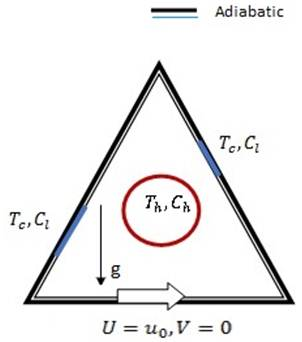Fig. 1. Schematic diagram of the triangular cavity.

The physical system considered in the present study is presented in the fig. 1. It is a two dimensional triangular enclosure with a heated obstacle in the middle of the triangular. The length and height of the enclosure are depicted by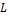and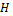respectively. The bottom wall is moves to the positive x-direction. The circular obstacle has the high temperature and high concentration. An external flow with low temperature and low concentration enters the middle-lower of the left wall and middle-upper to the right wall. All other walls are assumed to be adiabatic. The diameter of the circular obstacle is kept at 0.4 in this work. The inlet size of both the wall is equal to one fifth of the enclosure length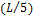. All solid boundaries are assumed to be rigid and no-slip walls.

3. Governing Equations

The governing equations are based on the conservation of mass, momentum, energy and species concentration. The density in the buoyancy force term of the y-momentum equation follows the Boussinesq approximation. The detailed dimensionless governing equations can be written as following: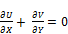(1)(2)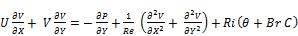(3)(4)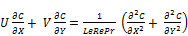(5)

where the dimensionless variables are defined as: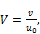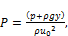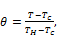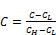(6)

and the five dimensionless parameters, Reynolds number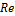, Prandtl number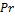, Richardson number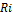, Lewis numberand buoyancy ratio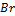, are: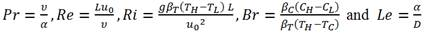(7)

The Richardson number, characterizes the relative importance of natural to forced convections. In this study,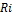is set to be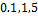and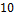representing the natural convection to forced convection. Lewis number, reflects the mass transfer exchange in the whole enclosure. The buoyancy ratio, are also studied with different values to consider the effect of double diffusive mixed convection. Prandtl number, is taken 0.1 for air and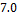for water. The dimensionless boundary conditions are specified as follows:

On the middle-lower left wall: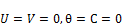(8a)

On the middle-upper right wall:(8b)

On the heated obstacle: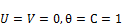(8c)

On the bottom wall: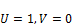(8d)

On all solid cavity walls: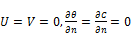(8e)

where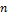is the non-dimensional distances either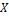or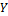direction acting normal to the surface.

The average nusselt number and sherwood numbers evaluated along the circular obstacle of the cavity based on the dimensionless quantities may be expressed as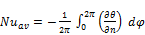(9)

and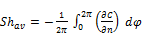(10)

Table 1. Grid sensitivity check at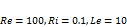and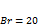.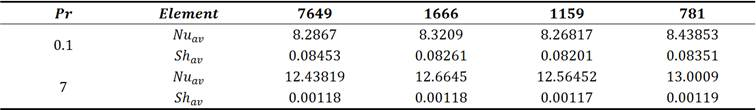4. Numerical Treatment

Finite element method has been used to solve Eqs.with the boundary conditions of Eqs.numerically. The numerical method used in this study is based on the finite element method to discretize the governing equations (Eqs.) and the set of algebraic equations are solved using a stationary non-linear together with direct linear system solver. In the case of all dependent variables, the relative tolerance for the error criteria was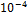. Non-uniform triangular grid system is engaged in this study. The Nusselt and Sherwood numbers for different grid sizes (781 to 7649) are presented to develop an understanding of the grid fineness that is necessary for accurate numerical simulation (Table 1). There is considerable change in Nusselt number for different values of Prandtl number with different grid sizes and no considerable change found in Sherwood number for different values of Prandtl number but changes occurs for different grid size.

5. Program Validation

Simulations were carried out to study the double-diffusive mixed convection in an open enclosure with different cylinder locations . Here an external flow with low temperature and concentration enters the lower-left wall and there is an exit at the upper-right wall. Heated and concentrated cylinder is placed on the middle of the enclosure. Rest of the walls are assumed to be adiabatic. All solid boundaries are assumed to be rigid and no-slip condition. Figs. 2 and 3 show the comparison of the predicted results of isoconcentration, streamline and isotherm by this program with the result from Xu et al. .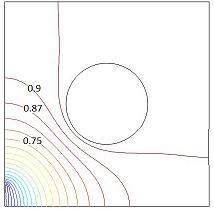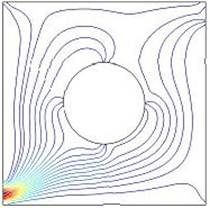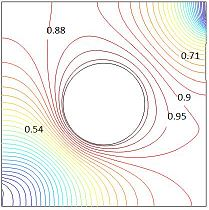Fig. 2. Isoconcentration, streamline and isotherm atand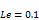(present).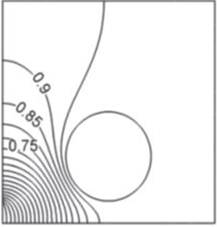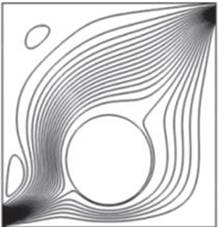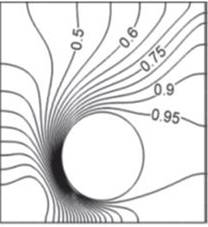Fig. 3. Isoconcentration, streamline and isotherm atand(Xu et al. ).

6. Results and Discussion

A numerical simulation has been done by using finite element method and has investigated the mixed convection heat and mass transfer in a lid-driven triangular enclosure having a heated obstacle. Two cases were considered and tested according to different values of Prandtl number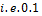for air andfor water respectively. Here the governing parameter affecting heat transfer is the Richardson number. For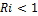, the flow and heat transfer is dominated by forced convection, for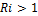, it is dominated by natural convection and for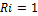, it is a mixed regime. Computations are chosen for different parameters such as Prandtl number,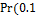and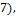the Reynolds number,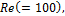buoyancy ratio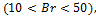Lewis number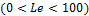and Richardson number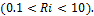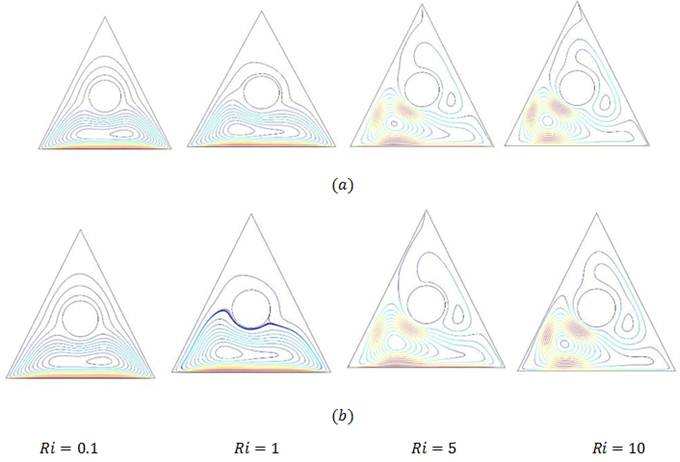Fig. 4. Effect of Richardson number on streamlines for (a) case 1 and (b) case 2 with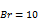.

Fig. 4. shows the effect of Richardson number on streamlines for two different cases as case 1 (for air with) and case 2 (for water with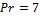) at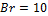. Here the lid moves to the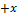direction and fluid inside the cavity impinges to the left corner of the triangular cavity with the increase ofand it circulates in clockwise direction for both cases. However, atthe top corner of the triangular cavity becomes stagnant and there is no other circulation cell inside the cavity with the increases of Richardson number. As seen from the figures, effects of Lewis number become insignificant on the flow field inside the cavity. Again fig. 7. shows the effect of buoyancy ratio on streamlines for case1 and case 2 with Ri=5. These shows that with the increase of buoyancy ratio circulation can be formed within the right wall included the circular obstacle. At low Prandtl number no circulation can be twisted but at high Prandtl number circulation becomes twisted and and another circulation found at the right corner of the triangular cavity. Also Lewis number is not effective for both cases.

Effect of Richardson number on isotherm are presented in fig. 5. for both case. The cavity is heated due to the circular obstacle in the middle but is suppressed by moving lid in the +x direction. Isotherms are clustered near the left lower middle and the right upper middle wall respectively. At low Prandtl number with the increase of Ri, heat can flows all over the cavity. But at high Prandtl number the right corner of the cavity become stagnant and no heat can flows in that region. Also heat flows around the circular obstacle and reaches slowly left to top corner of the cavity with the increase of Ri at High Prandtl number. Fig. 8. shows the effect of buoyancy ratio on isotherms for both cases. These figures shows that what happens if Br varies from 10 to 50. Here we observed that with increase of buoyancy ratio heat moves from top to right wall i.e. it moves in clockwise direction at high Prandtl number. Whereas at low Prandtl number the changes in isotherms are negligible.

Isoconcentration contours are shown in fig. 6. and fig. 9. Fig. 6. illustrates that at low Richardson number there is no change in isoconcentration for both cases, but at high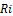there is a little bit change. Fig. 9. shows that with the increase of buoyancy ratio isoconcentration are distributed from circular obstacle to the right inclined wall.

Heat and mass transfer are presented graphically in fig. 10. It shows that with increase of Richardson number Nusselt number increases. But at low Prandtl number this increasing graph is negligible whereas at high Prandtl number this increasing graph is noticeable. On the other hand at high Prandlt number Sherwood number become constant with the increase of Lewis number. But at high Prandtl number Sherwood number decreases with the increase of Lewis number.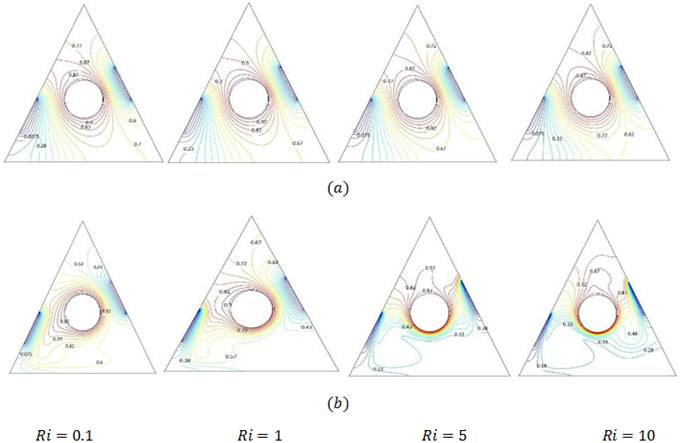Fig. 5. Effect of Richardson number on isotherm for (a) case 1 and (b) case 2 with.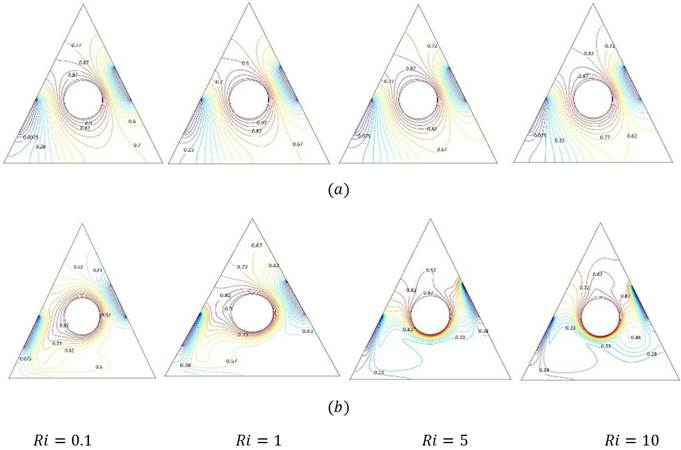Fig. 6. Effect of Richardson number on isoconcentration for (a) case 1 and (b) case 2 with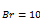.Fig. 7. Effect of buoyancy ratio on streamlines for (a) case 1 and (b) case 2 with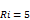.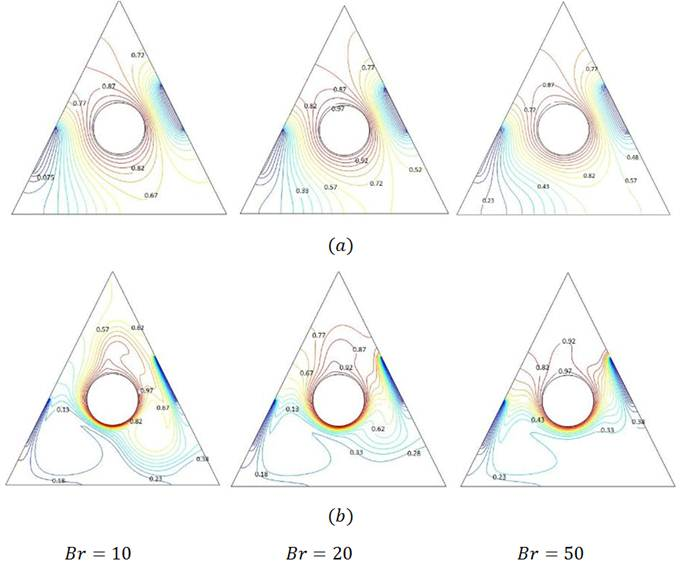Fig. 8. Effect of buoyancy ratio on isotherms for (a) case 1 and (b) case 2 with.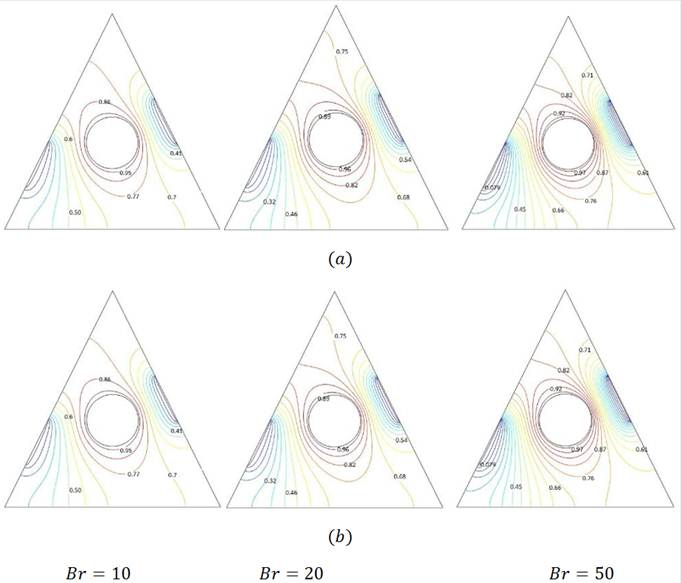Fig. 9. Effect of buoyancy ratio on isoconcentration for (a) case 1 and (b) case 2 with.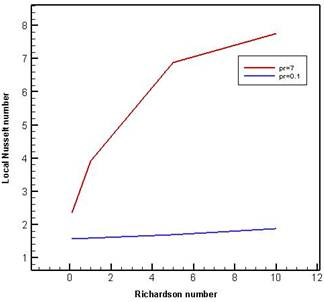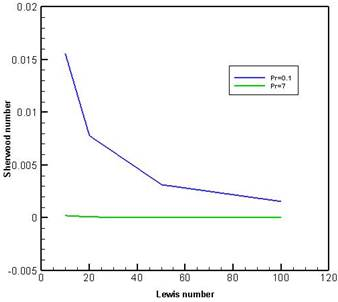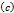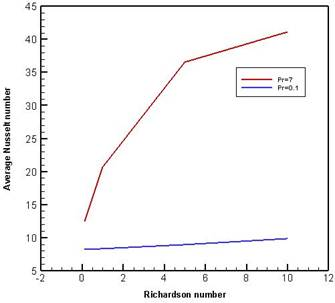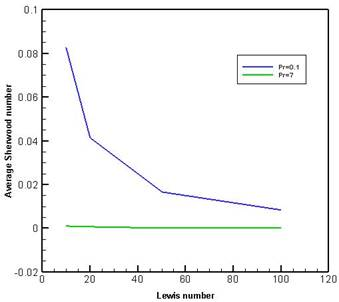Fig. 10. (a) Local Nusselt number versus Ri (b) Average Nusselt number versus Ri (c) Sherwood number versus Le (d) Average Sherwood number versus Le for both cases.

7. Conclusion

A computational work has been performed to study the effects of Richardson number and buoyancy ratio on heat and mass transfer in a triangular cavity with heated obstacle and right and left inlet cold wall for different values of Prandtl number. Some important findings can be listed as follows:

Heat transfer increases with the increase of Richardson number. At high Prandtl number heat transfer rate increases rapidly with the increase of Richardson number. But at low Prandtl number heat transfer rate increases linearly with the increase of Richardson number.

Sherwood number decreases with the increase of Lewis number. At low Prandtl number Sherwood number decrease rapidly with the increase of Lewis number. But at high Prandtl number Sherwood number moves constantly with the increase of Lewis number.

Buoyancy Ratio plays an important role on temperature distribution. Also it plays important role for high Prandtl number than low Prandtl number.

Lewis number becomes insignificant on the flow field and temperature distribution. However, Lewis number is an effective parameter on isoconcentration.

Nomenclature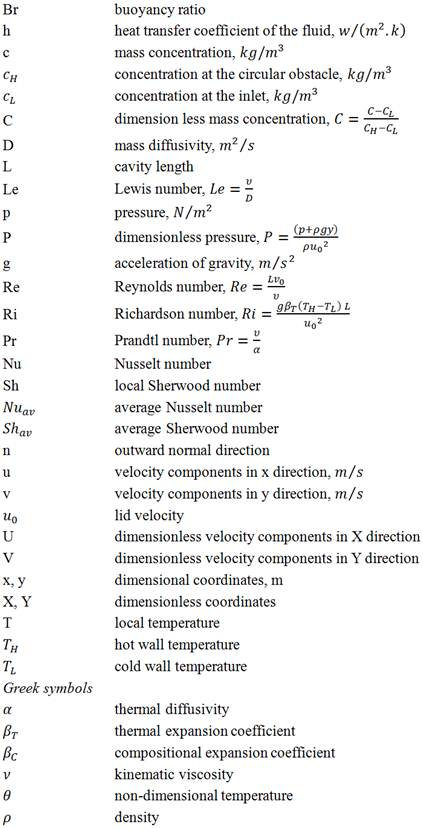References

1. M. Morzynski, C.O. Popiel, Laminar heat transfer in a two-dimensional cavity covered by a moving wall, Numer. Heat Transfer 12 (1988) 265–273.
2. M.K. Moallemi, K.S. Jang, Prandtl number effects on laminar mixed convection heat transfer in a lid-driven cavity, Int. J. Heat Mass Transfer 35 (1992) 1881–1892.
3. R. Iwatsu, J.M. Hyun, K. Kuwahara, Mixed convection in a driven cavity with a stable vertical temperature gradient, Int. J. Heat Mass Transfer 36 (1993) 1601–1608.
4. A.K. Prasad, J.R. Koseff, Combined forced and natural convection heat transfer in a deep lid-driven cavity flow, Int. J. Heat Fluid Flow 17 (1996) 460–467.
5. H. Asan, L. Namli, Laminar natural convection in a pitched roof of triangular cross-section: summer day boundary conditions, Energy and Buildings 33 (2000) 69–73.
6. G.A. Holtzman, R.W. Hill, K.S. Ball, Laminar natural convection in isosceles triangular enclosures heated from below and symmetrically cooled from above, J. Heat Transfer 122 (2000) 485–491.
7. V.A. Akinsete, T.A. Coleman, Heat transfer by steady laminar free convection in triangular enclosures, Int. J. Heat Mass Transfer 25 (1982) 991–998.
8. H. Salmun, Convection patterns in a triangular domain, Int. J. Heat Mass Transfer 38 (1995) 351–362.
9. A. Koca, H. F. Oztop, Y. Varol, The effects of Prandtl number on natural convection in triangular enclosure with localized heating from below, Int. Commun. Heat Mass Transfer 34 (2007) 511-519.
10. M.A. Teamah, A.F. Elsafty, E.Z. Massoud, Numerical simulation of double-diffusive natural convective flow in an inclined rectangular enclosure in the presence of magnetic field and heat source, Int. J. Therm. Sci. 52 (2012) 161–175.
11. H. T. Xu, Z. Y. Wang, F. Karimi, M. Yang, Y. W. Zhang, Numerical simulation of double diffusive mixed convection in an open enclosure with different cylinder locations, Int. Commun. Heat Mass Transfer 52 (2014) 33-45.
12. M. M. Rahman, H. F. Oztop, A. Ahsan, J. Orfi, Natural convection on heat and mass transfer in a curvilinear triangular cavity, Int. J. Heat Mass Transfer 55 (2012) 6250–6259.
13. M. Hasanuzzamzn, M. M. Raahman, H.F. Oztop, N.A. Rahim, R. Saidur, Effects of Lewis number on heat and mass transfer in a triangular cavity, Int. Commun. Heat Mass Transfer 39 (2012) 1213-1219.
14. Khalil Khanafer, Comparison of flow and heat transfer characteristics in a lid-driven cavity between flexible and modified geometry of a heated bottom wall, Int. J. Heat Mass Transfer 78 (2014) 1032–1041.
15. Y. C. Ching, H. F. Oztop, M. M. Rahman, M. R. Islam, A. Ahsan, Finite element simulation of mixed convection heat and mass transfer in a right triangular enclosure, Int. Commun. Heat Mass Transfer 39 (2012) 689-696.
16. S. Parvin, N. F. Hossain, Finite element simulation of MHD combined convection through a triangular wavy channel, Int. Commun. Heat Mass Transfer 39 (2012) 811-817.
17. M. M. Rahman, M. A. Alim, M. M. A. Sarker, Numerical study on the conjugate effect of joule heating and magneto-hydrodynamics mixed convection in an obstructed lid-driven square cavity, Int. Commun. Heat Mass Transfer 37 (2010) 524-534.
18. K. Al-Salem, H. F. Öztop, I. Pop, Y. Varol, " Effects of moving lid direction on MHD mixed convection in a linearly heated cavity", Int. J. Heat Mass Transfer 55 (2012) 1103–1112.
19. A. Al-Amiri, K. Khanafer, J. Bull, I. Pop, "Effect of sinusoidal wavy bottom surface on mixed convection heat transfer in a lid-driven cavity", Int. J. Heat Mass Transfer 50 (2007) 1771–1780.
20. R. Chowdhury, M. A. H Khan, M. N. A. Siddiki, Natural convection in porous triangular enclosure with a circular obstacle in presence of heat generation, Amer. J. App. Math. 3 (2015) 51-58.
21. S.V. Patankar, Numerical Heat Transfer and Fluid Flow, Hemisphere McGraw-Hill, Washington DC, 1980.

 Contents 1. 2. 3. 4. 5. 6. 7.
Article ToolsAbstractPDF(4103K)